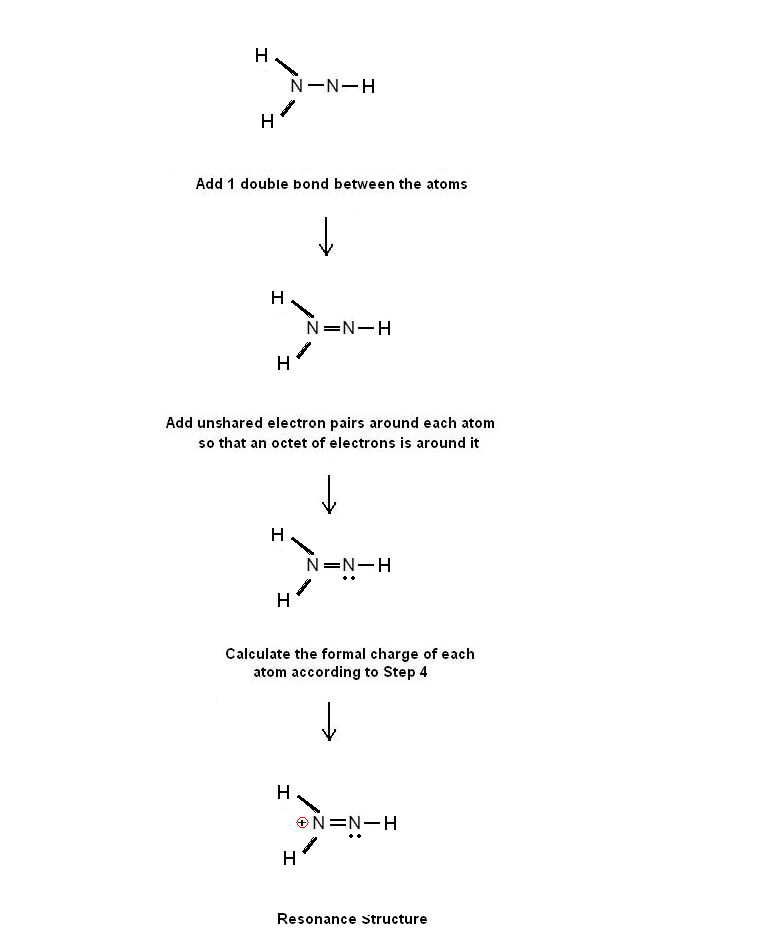Simple Procedure for writing Dot Structures – # 26 - Diazenium, N2H3+ | Chemistry Net

# Simple Procedure for writing Dot Structures – # 26 - Diazenium, N2H3+

A simple procedure for writing Lewis electron structures is given in a previous article entitled “Lewis Structures and the Octet Rule”.
Several worked examples relevant to this procedure were given in previous posts please see the Sitemap - Table of Contents (Lewis Electron Dot Structures).

Let us consider the case of  diazenium ion, N2H3+.

Step 1: Connect the atoms with single bonds. The central atom is the nitrogen atom.Fig. 1: Connect the atoms of the diazenium ion N2H3+ with single bonds.

Step 2: Calculate the # of electrons in π bonds (multiple bonds) using  formula (1)

Where n in this case is 5 since N2H3+ consists of 5 atoms.
Where V = (5 + 5 +1 + 1 + 1 ) -1 = 12
Therefore, P = 6n + 2 – V = 6 *2 + 2 – 12 = 2   Therefore, there is one double bond in the structure in Step 1.

Step 3 & 4: The Lewis structure for N2H3+  is as follows:Figure 2: Lewis structure for diazenium ion